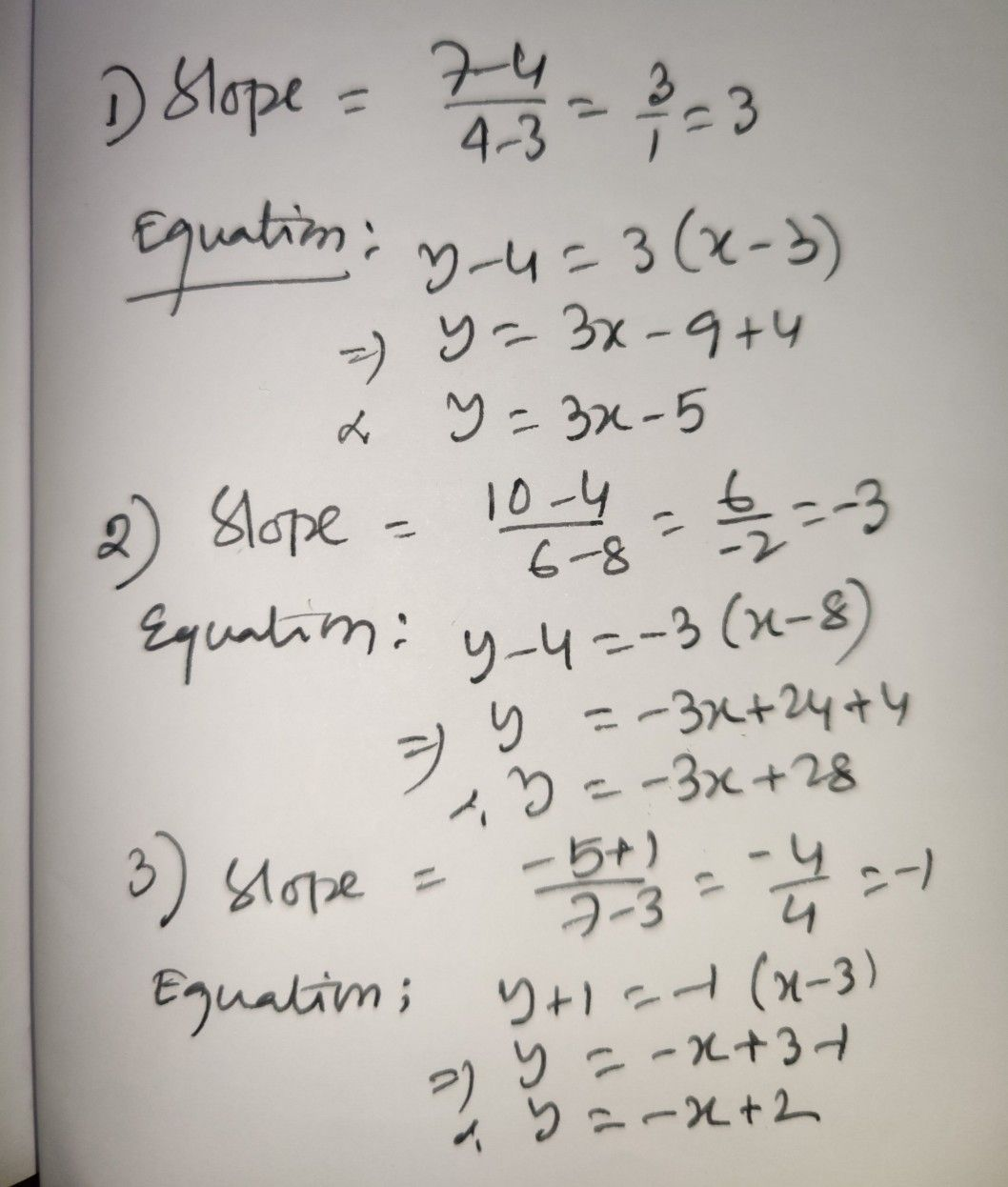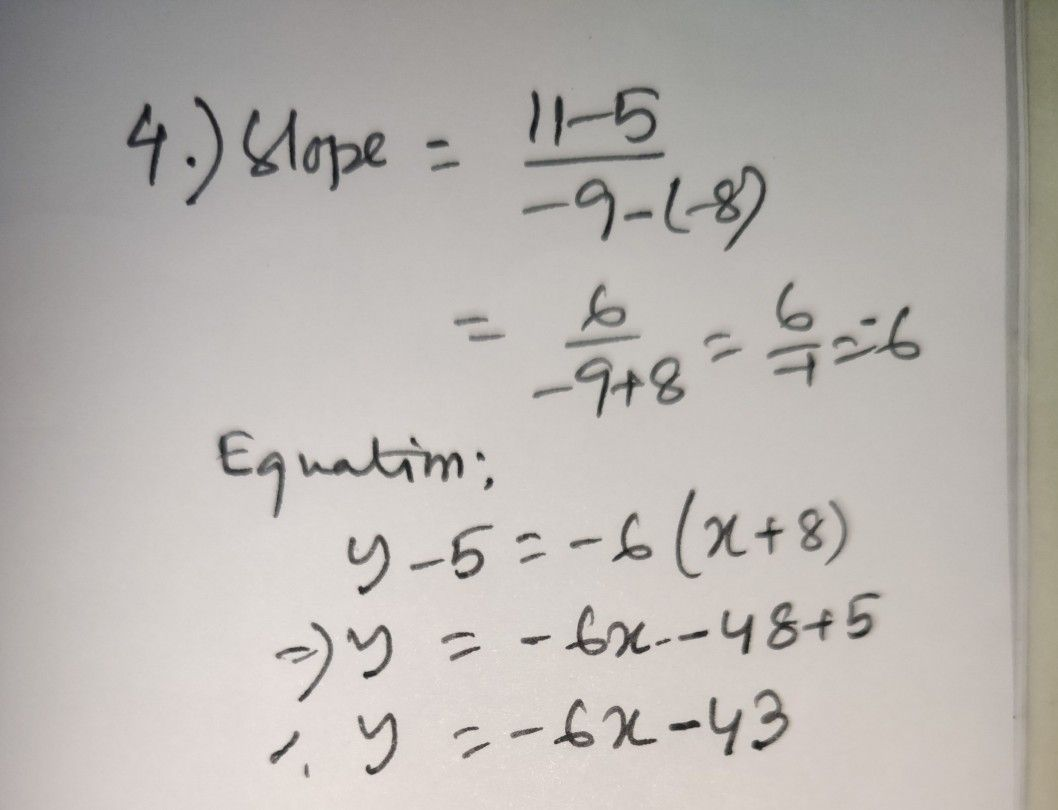Symbol
ProblemExercise 1. Find the equation $of$ the line of the form $y=mx+b$ that passes through the following pairs $o$ points. $\left(Use$ separate sheet $fory$ your answers.) $S.\right)$ $1.\right)$ $\left(3,4\right)and\left(4,$ $7\right)$ $10\right)$ $2.\right)$ $\left(8$ $4\right)and\left(6$ $3.\right)$ $\left(3,-1\right)$ $-1\right)and\left(7,-5\right)$ $4.\right)$ $\left(-8$ $5\right)and\left(-9,11\right)$
10th-13th grade
Other
Search count: 106
SolutionQanda teacher - RaiKindly Evaluate the Answer if you're satisfied with my answer.